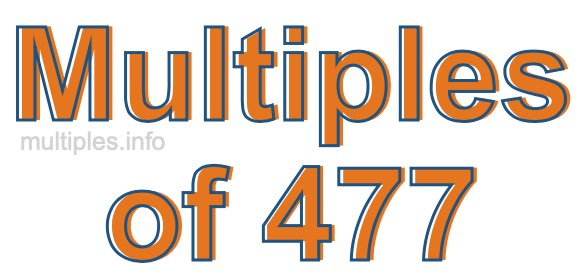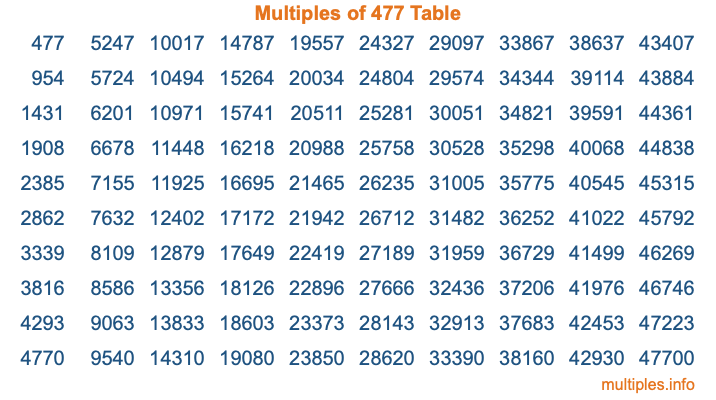Multiples of 477Welcome to the Multiples of 477 page. Here we will first teach you everything you will ever need to know about the multiples of 477, and then give you a study guide summary of everything we taught you to make sure you remember it all. Use this page to look up facts and learn information about the multiples of 477. This page will make you a multiples of four hundred seventy-seven expert!

Definition of Multiples of 477
Multiples of 477 are all the numbers that when divided by 477 equal an integer. Each of the multiples of 477 are called a multiple. A multiple of 477 is created by multiplying 477 by an integer.

Therefore, to create a list of multiples of 477, you start with 1 multiplied by 477, then 2 multiplied by 477, then 3 multiplied by 477, and so on for as long as you want. Thus, the list of the first five multiples of 477 is 477, 954, 1431, 1908, and 2385. To see a larger list of multiples of 477, see the printable image of Multiples of 477 further down on this page. We also have a category where you can choose any nth multiple of 477.

Multiples of 477 Checker
The Multiples of 477 Checker below checks to see if any number of your choice is a multiple of 477. In other words, it checks to see if there is any number (integer) that when multiplied by 477 will equal your number. To do that, we divide your number by 477. If the the quotient is an integer, then your number is a multiple of 477.

Is  a multiple of 477?

Least Common Multiple of 477 and ...
A Least Common Multiple (LCM) is the lowest multiple that two or more numbers have in common. This is also called the smallest common multiple or lowest common multiple and is useful to know when you are adding our subtracting fractions. Enter one or more numbers below (477 is already entered) to find the LCM.

Check out our LCM Calculator if you need more details about the Least Common Multiple or if you need the LCM for different numbers for adding and subtraction fractions.

nth Multiple of 477
As we stated above, 477 is the first multiple of 477, 954 is the second multiple of 477, 1431 is the third multiple of 477, and so on. Enter a number below to find the nth multiple of 477.

th multiple of 477

Multiples of 477 vs Factors of 477
477 is a multiple of 477 and a factor of 477, but that is where the similarities end. All postive multiples of 477 are 477 or greater than 477. All positive factors of 477 are 477 or less than 477.

Below is the beginning list of multiples of 477 and the factors of 477 so you can compare:

Multiples of 477: 477, 954, 1431, 1908, 2385, etc.

Factors of 477: 1, 3, 9, 53, 159, 477

As you can see, the multiples of 477 are all the numbers that you can divide by 477 to get a whole number. The factors of 477, on the other hand, are all the whole numbers that you can multiply by another whole number to get 477.

It's also interesting to note that if a number (x) is a factor of 477, then 477 will also be a multiple of that number (x).

Multiples of 477 vs Divisors of 477
The divisors of 477 are all the integers that 477 can be divided by evenly. Below is a list of the divisors of 477.

Divisors of 477: 1, 3, 9, 53, 159, 477

The interesting thing to note here is that if you take any multiple of 477 and divide it by a divisor of 477, you will see that the quotient is an integer.

Multiples of 477 Table
Below is an image of the first 100 multiples of 477 in a table. The table is in chronological order, column by column. The first column has the first ten multiples of 477, the second column has the next ten multiples of 477, and so on.The Multiples of 477 Table is also referred to as the 477 Times Table or Times Table of 477. You are welcome to print out our table for your studies.

Negative Multiples of 477
Although not often discussed or needed in math, it is worth mentioning that you can make a list of negative multiples of 477 by multiplying 477 by -1, then by -2, then by -3, and so on, to get the following list of negative multiples of 477:

-477, -954, -1431, -1908, -2385, etc.

Multiples of 477 Summary
Below is a summary of important Multiples of 477 facts that we have discussed on this page. To retain the knowledge on this page, we recommend that you read through the summary and explain to yourself or a study partner why they hold true.

There are an infinite number of multiples of 477.

A multiple of 477 divided by 477 will equal a whole number.

477 divided by a factor of 477 equals a divisor of 477.

The nth multiple of 477 is n times 477.

The largest factor of 477 is equal to the first positive multiple of 477.

477 is a multiple of every factor of 477.

477 is a multiple of 477.

A multiple of 477 divided by a divisor of 477 equals an integer.

477 divided by a divisor of 477 equals a factor of 477.

Any integer times 477 will equal a multiple of 477.

Multiples of a Number
Here you can get the multiples of another number, all with the same attention to detail as we did for multiples of 477 on this page.

Multiples of
Multiples of 478
Did you find our page about multiples of four hundred seventy-seven educational? Do you want more knowledge? Check out the multiples of the next number on our list!